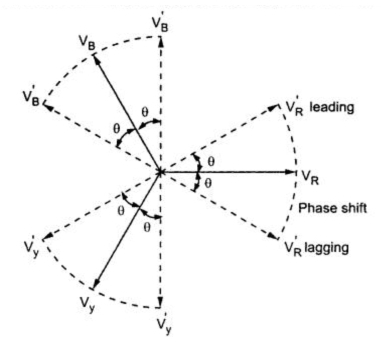Phase Shift Transformer

In this type of transformer, a laminated silicon steel stator consisting of either two phase or three phase winding similar to that in case of induction motor. A typical drysdale phase shifting transformer is shown in the Fig. 1.Fig. 1 Drysdale phase shifting transformer

The rotor winding is housed in the slots provided on laminated structure of the rotor. A small air gap is present between stator and rotor. The rotor can be moved from its initial zero position through a desired angle such that the equal phase displacement in rotor e.m.f. can be observed. With the help of pointer and scale provided at the top of the instrument, the angle through which the rotor has been moved can be indicated. The scale contains angles in degrees of phase displacement as well as cosines of these angles.
When stator winding is excited by voltage then rotating magnetic field is produced. This field gets linked with the rotor winding to induce e.m.f. in it. The windings on the rotor are arranged in such a way that change in magnitude of induced emf is not followed after the change in phase of this emf.
When the stator is excited from single phase supply as shown in the Fig. 1, then phase splitting device is to be used as rotating magnetic field is not produced by single phase supply. Thus two separate stator windings displaced from each other by an angle of 90o in space are used. The phase splitting device consists of a variable resistor and variable capacitor connected in series with one of the stator winding. The other winding is directly connected to the supply lines. The values of resistor and capacitor are successively adjusted till the currents in the two windings are displaced from each other by an angle of 90o for producing uniform rotating magnetic field. This is called quadrature adjustment.
1.1 Operation
The action of phase splitting device can be understood by considering the Fig. 2(a) and (b).Fig. 2

The corresponding phasor diagram is shown in the Fig. 3.Fig. 3

The circuit shown in the Fig. 2(b) is the equivalent circuit. When the currents are equal and quadrature ( 90o apart from each other) then we have,
I2 = j I1

Equating real and imaginary parts,

Note : Hence by adjusting the value of R and C, phase splitting is possible.
If we considering a particular position of the rotor then the induced emf in each of the two stator windings is proportional to the cosine of the angular displacement from the position of maximum emf.
Emf induced in rotor winding due to first stator winding is given by,
e1 = KI sinωt cosθ
Similarly emf induced in rotor winding due to second stator winding is given by,
e2 = KI sin (ωt + 90o) cos(θ + 90o)
= - KI cos ωt sinθ
The resultant emf in the rotor is given by,
e = e1 + e2 = KI sinωt cosθ - KI cosωt sinθ
= KI (sinωt cosθ - cosωt sinθ)
We have, sin (A-B) = sin A cos B - cos A sin B
...                  e = KI sin (ωt - θ)
From the above relation, it can be seen that the emf induced in the rotor has a constant amplitude but its phase depends on the position of rotor i.e. an angle θ.
With the availability of three phase supply, three phase shifting transformer can be used. It is less sensitive to frequency changes than the phase splitting circuit which uses single phase supply.
In case of three phase shift transformer the phase angle between incoming and outgoing lines is shifted without change in the voltage ratio. It is shown in the Fig. 4.Fig. 4 Phase shift transformer

The three phase lines is connected to incoming terminals of phase shifting transformer. The voltage at output terminals is displaced from incoming voltage by some angle without change in magnitude. Thus voltages at output terminals are displaced incoming voltages which is shown in the phasor diagram in the Fig. 5.Fig. 5 Phasor diagram

Note : The phase angle is sometimes varied in discrete steps by means of a motorized tap changer for precise control of shift.

Related Articles :